Updating search results...

# 10 Results

View
Selected filters:
• CCSS.Math.Content.7.SP.C.5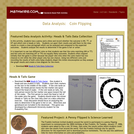Rating
0.0 stars

Using two different coins and recording the results of both coins helps students dispel this initial misconception as they analyze the graph results. Class discussion should focus on analyzing the data to determine if the game is fair or not. Directions and gameboard are included in the download.

Material Type:
Game
Provider:
Mathwire
Author:
Terry Kawas
02/16/2011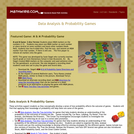Rating
0.0 stars

These activities support students as they conceptually develop a sense of how probability affects the outcome of games. Students will find that applying their knowledge of probability will help them win some of the games

Material Type:
Game
Provider:
Mathwire
Author:
Terry Kawas
02/16/2011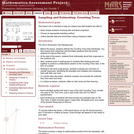Only Sharing Permitted
CC BY-NC-ND
Rating
0.0 stars

This lesson unit is intended to help you assess how well students are able to: solve simple problems involving ratio and direct proportion; choose an appropriate sampling method; and collect discrete data and record them using a frequency table.

Subject:
Education
Geometry
Mathematics
Measurement and Data
Ratios and Proportions
Material Type:
Assessment
Lecture Notes
Lesson Plan
Teaching/Learning Strategy
Provider:
Shell Center for Mathematical Education
Provider Set:
Mathematics Assessment Project (MAP)
Author:
http://map.mathshell.org/
04/26/2013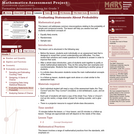Only Sharing Permitted
CC BY-NC-ND
Rating
0.0 stars

This lesson unit addresses common misconceptions relating to probability of simple and compound events. The lesson will help you assess how well students understand concepts of: Equally likely events; randomness; and sample sizes.

Subject:
Mathematics
Statistics and Probability
Material Type:
Assessment
Lesson Plan
Provider:
Shell Center for Mathematical Education
Provider Set:
Mathematics Assessment Project (MAP)
04/26/2013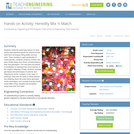Educational Use
Rating
0.0 stars

Students randomly select jelly beans (or other candy) that represent genes for several human traits such as tongue-rolling ability and eye color. Then, working in pairs (preferably of mixed gender), students randomly choose new pairs of jelly beans from those corresponding to their own genotypes. The new pairs are placed on toothpicks to represent the chromosomes of the couple's offspring. Finally, students compare genotypes and phenotypes of parents and offspring for all the "couples" in the class. In particular, they look to see if there are cases where parents and offspring share the exact same genotype and/or phenotype, and consider how the results would differ if they repeated the simulation using more than four traits.

Subject:
Applied Science
Engineering
Genetics
Life Science
Material Type:
Activity/Lab
Lesson Plan
Provider:
TeachEngineering
Provider Set:
TeachEngineering
Author:
Mary R. Hebrank
09/26/2008Conditional Remix & Share Permitted
CC BY-NC
Rating
0.0 stars

Four full-year digital course, built from the ground up and fully-aligned to the Common Core State Standards, for 7th grade Mathematics. Created using research-based approaches to teaching and learning, the Open Access Common Core Course for Mathematics&nbsp;is designed with student-centered learning in mind, including activities for students to develop valuable 21st century skills and academic mindset.

Subject:
Mathematics
Material Type:
Full Course
Provider:
Pearson
10/06/2016Conditional Remix & Share Permitted
CC BY-NC
Rating
0.0 stars

Students choose a project idea and a partner or group. They write a proposal for their project.Key ConceptsProjects engage students in the applications of mathematics. It is important for students to apply mathematical ways of thinking to solve rich problems. Students are more motivated to understand mathematical concepts if they are engaged in solving a problem of their own choosing. In this lesson, students are challenged to identify an interesting mathematical problem and to choose a partner or a group to work collaboratively on solving that problem. Students gain valuable skills in problem solving, reasoning, and communicating mathematical ideas with others.Goals and Learning ObjectivesIdentify a project idea.Identify a partner or group to work collaboratively on a math project.

Subject:
Mathematics
Material Type:
Lesson Plan
09/21/2015Conditional Remix & Share Permitted
CC BY-NC
Rating
0.0 stars

Samples and ProbabilityType of Unit: ConceptualPrior KnowledgeStudents should be able to:Understand the concept of a ratio.Write ratios as percents.Describe data using measures of center.Display and interpret data in dot plots, histograms, and box plots.Lesson FlowStudents begin to think about probability by considering the relative likelihood of familiar events on the continuum between impossible and certain. Students begin to formalize this understanding of probability. They are introduced to the concept of probability as a measure of likelihood, and how to calculate probability of equally likely events using a ratio. The terms (impossible, certain, etc.) are given numerical values. Next, students compare expected results to actual results by calculating the probability of an event and conducting an experiment. Students explore the probability of outcomes that are not equally likely. They collect data to estimate the experimental probabilities. They use ratio and proportion to predict results for a large number of trials. Students learn about compound events. They use tree diagrams, tables, and systematic lists as tools to find the sample space. They determine the theoretical probability of first independent, and then dependent events. In Lesson 10 students identify a question to investigate for a unit project and submit a proposal. They then complete a Self Check. In Lesson 11, students review the results of the Self Check, solve a related problem, and take a Quiz.Students are introduced to the concept of sampling as a method of determining characteristics of a population. They consider how a sample can be random or biased, and think about methods for randomly sampling a population to ensure that it is representative. In Lesson 13, students collect and analyze data for their unit project. Students begin to apply their knowledge of statistics learned in sixth grade. They determine the typical class score from a sample of the population, and reason about the representativeness of the sample. Then, students begin to develop intuition about appropriate sample size by conducting an experiment. They compare different sample sizes, and decide whether increasing the sample size improves the results. In Lesson 16 and Lesson 17, students compare two data sets using any tools they wish. Students will be reminded of Mean Average Deviation (MAD), which will be a useful tool in this situation. Students complete another Self Check, review the results of their Self Check, and solve additional problems. The unit ends with three days for students to work on Gallery problems, possibly using one of the days to complete their project or get help on their project if needed, two days for students to present their unit projects to the class, and one day for the End of Unit Assessment.

Subject:
Mathematics
Statistics and Probability
Material Type:
Unit of Study
Provider:
PearsonConditional Remix & Share Permitted
CC BY-NC
Rating
0.0 stars

Students begin to formalize their understanding of probability. They are introduced to the concept of probability as a measure of likelihood and how to calculate probability as a ratio. The terms discussed (impossible, certain, etc.) in Lesson 1 are given numerical values.Key ConceptsStudents will think of probability as a ratio; it can be written as a fraction, decimal, or a percent ranging from 0 to 1.Students will think about ratio and proportion to predict results.Goals and Learning ObjectivesDefine probability as a measure of likelihood and the ratio of favorable outcomes to the total number of outcomes for an event.Predict results based on theoretical probability using ratio and proportion.

Subject:
Statistics and Probability
Material Type:
Lesson Plan
09/21/2015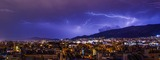Conditional Remix & Share Permitted
CC BY-NC
Rating
0.0 stars

Students will begin to think about probability by considering how likely it is that their house will be struck by lightning. They will consider the relative likelihood of familiar events (e.g., outdoor temperature, test scores) on the continuum between impossible and certain. Students will discuss where on the continuum &quot;likely,&quot; &quot;unlikely,&quot; and &quot;equally likely as unlikely&quot; are.Key ConceptsAs students begin their study of probability, they look at the likelihood of events. Students have an intuitive sense of likelihood, even if no numbers or ratios are attached to the events. For example, there is clearly a better chance that a specific student will be chosen at random from a class than from the entire school.Goals and Learning ObjectivesThink about the concept of likelihood.Understand that probability is a measure of likelihood.Informally estimate the likelihood of certain events.Begin to think about why one event is more likely than another.SWD: Students with disabilities may need additional support seeing the relationships among problems and strategies. Throughout this unit, keep anchor charts available and visible to assist them in making connections and working toward mastery. Provide explicit think alouds comparing strategies and making connections. In addition, ask probing questions to get students to articulate how a peer solved the problem or how one strategy or visual representation is connected or related to another.

Subject:
Mathematics
Statistics and Probability
Material Type:
Lesson Plan